# Criteria for Congruence of Triangles - ASA Congruence Criterion

#### definition

ASA Congruence criterion: If under a correspondence, two angles and the included side of a triangle are equal to two corresponding angles and the included side of another triangle, then the triangles are congruent.

# ASA Congruence criterion:

If under a correspondence, two angles and the included side of a triangle are equal to two corresponding angles and the included side of another triangle, then the triangles are congruent.

Which congruence criterion do you use in the following?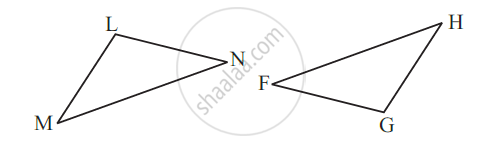Given:
∠MLN = ∠FGH
∠NML = ∠GFH
ML = FG
So, ∆LMN ≅ ∆GFH

Solution: ASA Congruence criterion, as two angles and the side included between these angles of ΔLMN, are equal to two angles and the side included between these angles of ΔGFH.

#### Example

By applying the ASA congruence rule, it is to be established that ∆ABC ≅ ∆QRP and it is given that BC = RP. What additional information is needed to establish the congruence?

For the ASA congruence rule, we need the two angles between which the two sides BC and RP are included. So, the additional information is as follows:
∠B = ∠R and ∠C = ∠P.

#### Example

In Fig, can you use the ASA congruence rule and conclude that ∆AOC ≅ ∆BOD?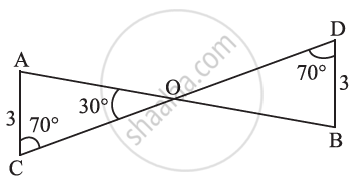In the two triangles AOC and BOD, ∠C = ∠D..................(each 70° )

Also, ∠AOC = ∠BOD = 30°........................(vertically opposite angles)

So, ∠A of ∆AOC =180° – (70° + 30°) = 80°..................(using angle sum property of a triangle)

Similarly, ∠B of ∆BOD =180° – (70° + 30°) = 80°

Thus, we have ∠A =∠B, AC = BD, and ∠C = ∠D

Now, side AC is between ∠A and ∠C and side BD is between ∠B and ∠D.

So, by ASA congruence rule, ∆AOC ≅ ∆BOD.

#### Example

Given below are measurements of some parts of two triangles. Examine whether the two triangles are congruent or not, by the ASA congruence rule. In the case of congruence, write it in symbolic form.

∆DEF, ∠D = 60º, ∠F = 80º, DF = 6 cm.

∆PQR, ∠Q = 60º, ∠R = 80º, QP = 6 cm.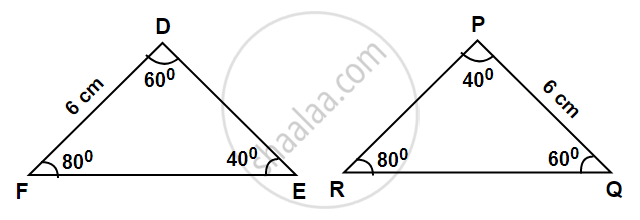∠D = ∠Q

∠F = ∠R

Side DF = Side PQ

So, ∆DEF ≅ ∆PQR.......(ASA congruence criterion)

#### theorem

Theorem (ASA congruence rule) :  Two triangles are congruent if two angles and the included side of one triangle are equal to two angles and the included side of other triangle.
Proof : We are given two triangles ABC and DEF in which:
∠ B = ∠ E, ∠ C = ∠ F
and BC = EF
We need to prove that   ∆ ABC ≅ ∆ DEF
For proving the congruence of the two triangles see that three cases arise.

Case (i) : Let AB = DE in following fig.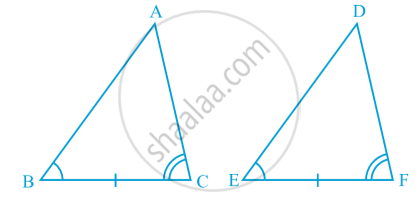You may observe that
AB = DE (Assumed)
∠ B = ∠ E (Given)
BC = EF (Given)
So, ∆ ABC ≅ ∆ DEF   (By SAS rule)

Case (ii) :
Let if possible AB > DE. So, we can take a point P on AB such that PB = DE. Now consider ∆ PBC and ∆ DEF  in following fig.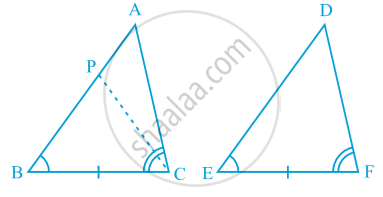Observe that in ∆ PBC and ∆ DEF,
PB = DE (By construction)
∠ B = ∠ E (Given)
BC = EF (Given)
So, we can conclude that:
∆ PBC ≅ ∆ DEF, by the SAS axiom for congruence.
Since the triangles are congruent, their corresponding parts will be equal.
So, ∠ PCB = ∠ DFE
But, we are given that
∠ ACB = ∠ DFE So, ∠ ACB = ∠ PCB
This is possible only if P coincides with A.
or, BA = ED
So, ∆ ABC ≅ ∆ DEF   (by SAS axiom)

Case (iii) :  If AB < DE, we can choose a point M on DE such that ME = AB and repeating the arguments as given in Case (ii),  we can conclude that AB = DE and so, ∆ ABC ≅ ∆ DEF.
You know that the sum of the three angles of a triangle is 180°. So if two pairs of angles are equal, the third pair is also equal (180° – sum of equal angles).
So, two triangles are congruent if any two pairs of angles and one pair of corresponding sides are equal. We may call it as the AAS Congruence Rule.

If you would like to contribute notes or other learning material, please submit them using the button below.

### Shaalaa.com

ASA Congruence Criterion [00:05:09]
S
0%